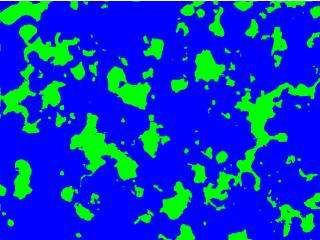-=+=- -=+=- -=+=- -=+=- -=+=- -=+=- -=+=- -=+=- -=+=- -=+=- -=+=- -=+=- -=+=- -=+=- -=+=- -=+=- -=+=- -=+=- -=+=- -=+=- -=+=- -=+=- -=+=- -=+=- -=+=- -=+=- -=+=- -=+=- -=+=- -=+=- (c) WidthPadding Industries 1987 0|469|0 -=+=- -=+=- -=+=- -=+=- -=+=- -=+=- -=+=- -=+=- -=+=- -=+=- -=+=- -=+=- -=+=- -=+=- -=+=- -=+=- -=+=- -=+=- -=+=- -=+=- -=+=- -=+=- -=+=- -=+=- -=+=- -=+=- -=+=- -=+=- -=+=- -=+=-

Jayenkai24th April 2019

# Ups

What did you get done this Month?
• Shoebox of Games
• Mostly coped with a day out.

Did everything turn out as planned?
No, the day out was almost a complete disaster when my head decided to crap out, midway, and I colla<snip>

• Jayenkai# JNKsg Template

8th April 2019

You can download the template here.
socoder.net/uploads/1/JNKsg_template.zip

A while back I created this little "app" to generate sprites using simple Javascript.

Every <snip>

Pakz# Additive random line Texture

6th April 2019Create a greyscale stone like texture

steve_ancell# Hacking the 74HC595 shift register.

31st March 2019

The 74HC595 is quite a handy chip for expanding the amount of outputs from a microcontroller, and can also be used to drive 7-segment number displays. This chip takes an 8-Bit binary stream and then outputs in parallel to the eight output pins. The 74HC165<snip>

Jayenkai# Taking a Longer Path

21st March 2019

Made a brute-force thing..

[code] Graphics 512,512,0,2
SetBuffer BackBuffer()

Global GridW=16
Global GridH=16
Global zx=512/GridW
Global zy=512/GridH

Global PlayerX,PlayerY,TargetX,TargetY,PathLength,Threshold

Dim grid(GridW,GridH)
Dim pa<snip>

spinal# Taking a Longer Path

21st March 2019

I was thinking something along these lines...

[code] Graphics 320,12,32,2

time1=MilliSecs()
a0=0:a1=0:a2=0:a3=0:a4=0:a5=0:a6=0:a7=0:a8=0:a9=0
fileout=WriteFile("moves.txt")

While a9<>3
a0=a0+1
If a0=4 Then a0=0:a1=a1+1
If a1=<snip>

spinal# Amiga Mouse

19th March 2019

All, but the original 500 mouse looked better and with those flat sides, would be easy to glue things to :-p

The Amiga mouse just passes all of the raw inputs directly to the computer for processing. It's really very similar to the 8-bit world of in<snip>

Jayenkai# What Have You Done - February 2019

27th February 2019

# Ups

What did you get done this Month?
• Started a Javascript board/card game engine
• Baked a cake
• Not much else, if I'm honest..

Did everything turn out as planned?
I guess it did. I'd've preferred a couple<snip>

• spinal# Making Universal Tools

3rd February 2019

Finished (enough for now) converter...

Will take an 8bit mono wav as input and turn it into an array that includes the samplerate and size.

[code]
<html>
<title>Wav to Pokitto</title>
<style type="text/css">
<snip>

spinal# Making Universal Tools

2nd February 2019

Here's a start, removed the output file for now, stuck in a txt box. I think it should do the job just right!

[code]
<html>
<style type="text/css">
body {background:#BB9977; color:#000000; font-family: "Helvetica<snip>

Jayenkai# Making Universal Tools

2nd February 2019

Convert a file using the browser

rychan# What Have You Done? - January 2019

29th January 2019

Apologies for posting this a little early but I felt the motivation to get this on here tonight for some reason# Ups

What did you get done in January 2019?
• Coded up the majority of a stock control system for work in .NET, works pr<snip>

• shroom_monk# *gfx++

28th January 2019

C++ operator precedence is well-defined, not compiler specific. See here: en.cppreference.com/w/cpp/language/operator_precedence. Though Jay's advice is still wise: it is often better for write your code more explicitly even if it(3)<snip>

spinal# *gfx++

28th January 2019

The odd thing I found with the above code, was that that was the only part of the function that seemed to break.

[code] void twoBitTile(int x1, int y1, const uint8_t *gfx){

int8_t wide = *gfx; // first byte is width
*gfx++;
int8_t high = <snip>

spinal# *gfx++

27th January 2019

So, why does this

work, but this
[code] int8_t wide = *gfx++; // first byte is width
int8_t high<snip>

Pakz# Bitwise technique

23rd January 2019

Id System for neighbouring tiles

Pakz# Maps or oilish paintings

21st January 2019Smearing a additive brush

Pakz# Pixel Art Pine Tree(s)

21st January 2019Generate sheets of pine trees(xmas?)

Jayenkai# What Have You Done - 2018

29th December 2018

# Ups

What did you get done in 2018?
• The 2018 Framework
• Foldapuz
• Online Sprite Generator
• A few games..

Did everything turn out as planned?
The framework seems to be running ok, and I even finally (over the past cou<snip>

• Jayenkai# Emscripten - Saving Data

23rd December 2018

*Saving an array of data, integers, binary, bytes, etc, into a single string, and storing it in localStorage, for the purpose of saving highscores, scores, progress, scoreboards, etc.*

This has NOT been an easy task!
I'm sure there's &qu<snip>

spinal# point between 2 numbers?

10th December 2018

[code] float spd = (POK_AUD_FREQ / sampleRates[channel]);
snd[channel].speed = (speed/spd); // recalculated above

// Default method, fast but awful.
ss[s] = snd[s].currentSound[(snd[s].soundPoint*snd[s].s<snip>

spinal# point between 2 numbers?

8th December 2018

either this isn't working, or it isn't the problme...
[code] // ss[s] = snd[s].currentSound[(snd[s].soundPoint*snd[s].speed)>>8];

int var1 = (snd[s].soundPoint*snd[s].speed);
int var2 = (snd[s].soundPoint*<snip>

Jayenkai# point between 2 numbers?

8th December 2018

Either of these help?

[code] Print "Percentage Between 10 and 70"
Print Perc(10,70,0)
Print Perc(10,70,50)
Print Perc(10,70,100)

Print
Print "Smooth gradual shift from 10 to 70"
a#=10
For n=0 To 10
Print a
a=Smooth(a,70<snip>

spinal# point between 2 numbers?

8th December 2018

I'm stuck on the last part of my sound player. I need to smooth out my wave form (in real time), currently I am re-sampling based on nearest-neighbour type algorithm.

[code]ss[s] = snd[s].currentSound[(snd[s].soundPoint*snd[s].speed)>>8];[/co<snip>

Jayenkai# Advent of Creativity - 2018

1st December 2018

Awesome!
I've attempted to learn Blender a few times, but I can never quite get the hang of it.
Good luck!

### Day Two

AL's posted this choon, today.
: Download
<snip>

More - Older Posts